# Object Localization with Classification in Real-Time

Object detection is one of the classical problems in the deep learning domain, where you work to recognize what and where the objects are in the image. Object detection is more complicated than classification, which can also identify the objects but doesn’t indicate where the object is. In the object detection system, we localize the object by putting the bounding box over it and the object’s class to it on the top of the bounding box, as shown in Figure 1.1.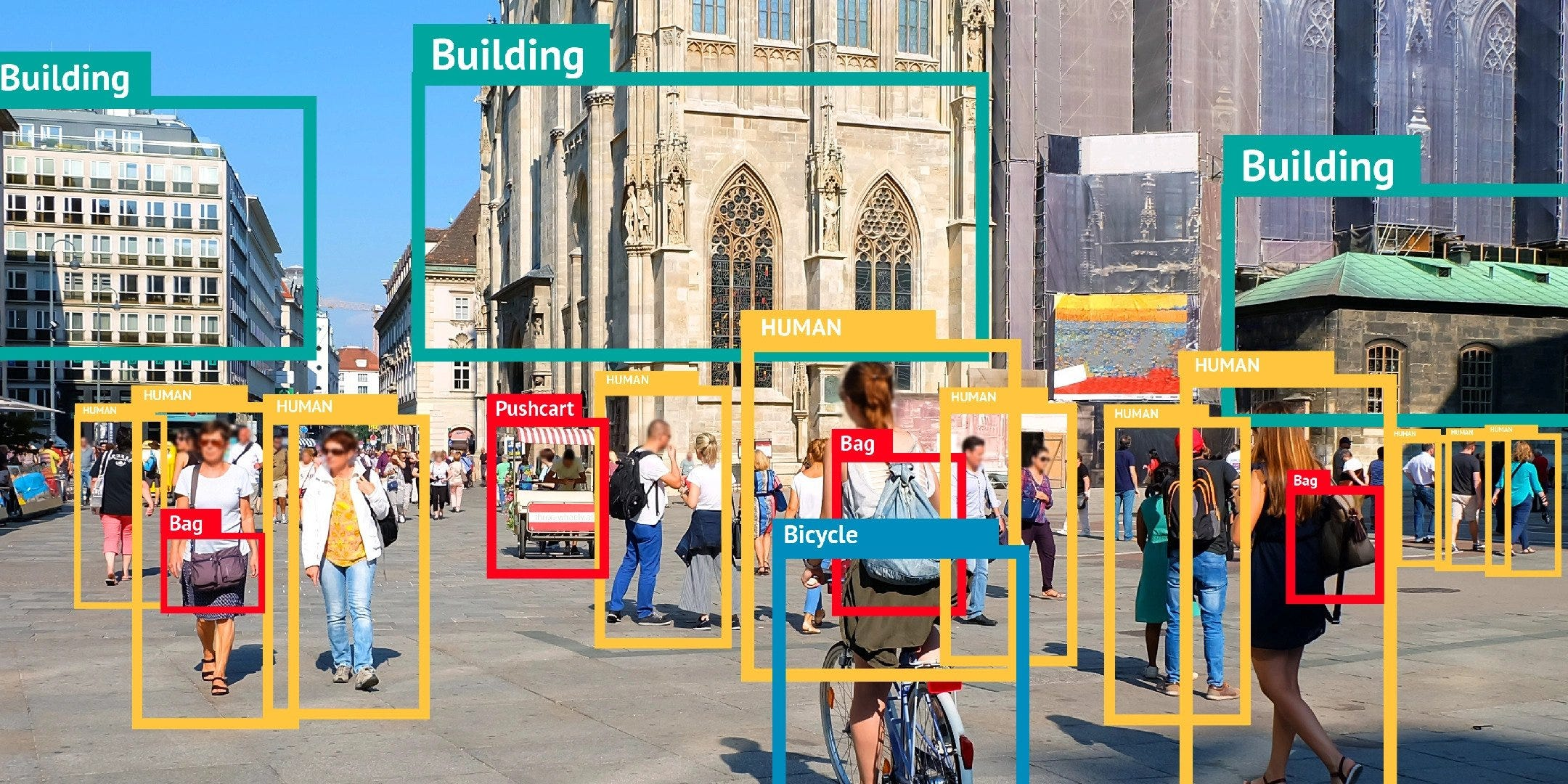Figure 1.1: Object Localization with Classification

There are several methods for object detection with classification, namely sliding window detection, convolutional implementation of the sliding window, R-CNN, YOLO, and many more.

Method 1: Sliding Window Detection

In the sliding window implementation, first, we have to train the convolutional neural network with the images of objects like cars, bicycles, humans, buildings, traffic signs, etc. We have to pick the rectangular region from the image. To pick the rectangular region, we have to set the hyperparameters like window size and stride. To select the best sliding window size with the best stride to cover the maximum number of objects in the image is not easy. If the window size is small, then we may miss large objects. If the window size is large, several objects may appear in one window, which is hard to detect because we can identify only one object per window. These rectangular regions are pass through the convolutional neural network model, which will predict the object with some probability.

Shortcomings of this method are computational cost, high object miss on the image, and significantly less accuracy. We can’t use this method in real-time.

Method 2: Convolutional Implementation of the Sliding Window

We can implement the sliding window method convolutionally; in this method, instead of passing selected rectangular windows of the image through the convolutional neural network model one at a time, it combines all the region into one form of computation and shares a lot of the computation in the region of the image that is common. Due to this, the computational cost decreases, but we may miss the objects on the image. This method’s accuracy is still low, and the inference time is better, but we can’t use it in real-time.

In Figure 1.2, Consider a 14X14 image that will pass through different layers of the model. On the bottom part of Figure 1.2, consider 16X16 image having four 14X14 patches if stride equals 2, instead of performing forward propagation on four portions of the input image separately. It combines all four into one form of computation and shares a lot of computation in the region of the image that is common.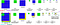Figure 1.2

Method 3: Region Based Convolutional Neural Networks

In Region-Based Convolutional Neural Networks, to avoid the problem of selecting a large number of regions in the given image, Ross Girshick et al. proposed a method in which we apply the selective search to extract just 2000 regions from the image, and this process is called region proposals. Therefore, instead of classifying a huge number of regions, we have to pass 2000 regions over CNN. To propose the region, we have to apply a selective search algorithm.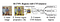Figure 1.3 

There are two up-gradation of RCNN, Fast RCNN, and Faster RCNN. However, the inference time of RCNN is much lesser than the sliding window algorithm, but in real-time applications can’t be used.

Method 4: You Only Look Once(YOLO)

You Only Look Once  is the state-of-the-art, real-time object detection system. In YOLO, we apply a single neural network to the full image. This network divides the image into regions and predicts bounding boxes and probabilities for each region. YOLO divides the images into 19X19 grid cells; only one grid cell is responsible for detecting one object where the object’s midpoint lies, as shown in Figure 1.4.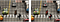Figure 1.4: Concept of the grid cell and midpoint

The output format of each grid cell is the column vector shown in the right part of Figure 1.5. The size of the vector is 5+number of classes where the 5 is pc, bx, by, bh, bw, and bx, by is the midpoint of the object and bh, bw are the height and width of the bounding box, and the number of classes is the number of objects our system can detect as shown in Figure 1.5.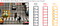Figure 1.5: Output Format

Model Output:

When we pass the image into the YOLO model, the model will give 1805 bounding boxes, as shown in Figure 1.6 with some objectness probability. To remove extra boxes, we use the non-max suppression algorithm.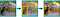Figure 1.6 Model Output 

Intersection Over Union(IOU):

Intersection over union is the concept used to find how much the rectangles overlap in the numeric form. The larger the value of IOU more is overlapping, and the more is redundancy. Thus, IOU can help in removing redundancy, and the non-max suppression algorithm uses it and explained in Figure 1.7, 1.8, 1.9.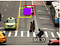Figure 1.7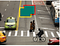Figure 1.8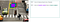Figure 1.9

Non-Maximum Suppression Algorithm:

Non-Maximum Suppression algorithm removes the unnecessary bounding boxes from the image. The result of this algorithm is the image having the necessary bounding boxes on it.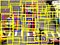Figure 1.10 Image with unnecessary bounding boxes

Algorithm:

1. Discard all the bounding boxes having pc < 0.6, as shown in Figure 1.11.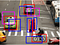Figure 1.11

2. While there are any remaining boxes:

• Pick the box having the largest pc as the prediction.
• Discard any remaining box with IOU ≥ 0.5 with the selected box in the previous step.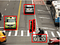Figure 1.12 Final output with necessary boxes

Anchor Boxes:

One of the problems with the previous version is that each grid cell can effectively detect one object. What if a midpoint of more than one object lies in one grid cell? as shown in Figure 1.13, where the car’s midpoint and the person’s midpoint lies in the same grid cell. We can use the idea of anchor boxes for solving this.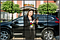Figure 1.13

Consider the sample image Figure 1.13, For that anchor boxes concept, is used, i.e., boxes of different sizes to detect both the object in one grid cell, as shown in Figure 1.14. YOLO uses five anchor boxes for one grid cell. Each anchor box is responsible for detecting one object in the grid cell. The anchor box who’s IOU is maximum with the object bounding box is considered as the reference anchor box for prediction of that object. Each object can be of different sizes and shapes. Therefore, the anchor boxes should also be of different sizes and shapes. The yellow anchor box predicts car while the red anchor box predicts person based on their shape.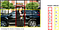Figure 1.14

Deciding the Number of Anchor Boxes:

To choose anchor boxes, YOLO uses the K-means clustering algorithm on the training data to locate the top K clusters’ centroids. Consider Figure 1.15 on the left, the plot of the average IOU between the anchors and the ground truth boxes using different numbers of clusters(anchors). As the number of anchors increases, the accuracy improvement is little. For the best return, YOLO settles down with five anchors. On the right, it displays the five anchor shapes. The purplish-blue rectangles are selected from the COCO dataset, while the black border rectangles are selected from the VOC 2007.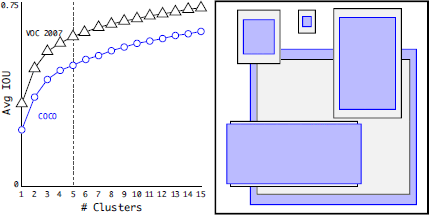Figure 1.15 

Result:

The line graph in Figure 1.16 shows the accuracy of different models when run on a COCO dataset. By analyzing the accuracy by mAP, we can see RetinaNet has better accuracy, but the mAP is not the only parameter to be considered. Since our focus is on real-time object detection, the other parameter has to be time. So, we need to establish a tradeoff between accuracy and time so that the model works efficiently when considered both. Thus, we can see the inference time for YOLO is minimum, considering the accuracy of 28.2–33.0. YOLO seems to be working better at a real-time system with degradation in the accuracy, which seems to be a better tradeoff with not much loss in the accuracy.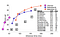Figure 1.16 

Implementation of YOLO:

Training:

YOLO training has major components dataset, data annotation, image preprocessing, data preprocessing, model, and loss function, as shown in Figure 1.17. The dataset should contain many training images and testing images of (height X weight) dimensioned. Each image of the dataset has annotations. Each annotation contains the rectangular bounding box coordinates, which are the coordinate of the upper left corner and lower right corner and the class. After getting the images and the annotations for the images, we will start the preprocessing. Preprocessing also has two parts first is image processing, and the second one is data processing. In the image processing, we reshape the image from (height X weight) into 608 X 608 to make it compatible with the model’s input. We have applied the normalized technique such that the pixel value will range from 0 to 1 to avoid the explosion of the gradient problem. In the data-preprocessing step, we will find the midpoint, height, and width of each bounding box using coordinates of the upper left corner and lower right corner of the rectangular box. The model’s input volume is 608 X 608 X 3, and the output is the 19 X 19 X 5 X (5+number of classes). To calculate the loss, we need to adjust the image’s annotation data into the YOLO output format. To do that, we are taking a detector mask of size 19 X 19 X 5 X 1. Its value is one when the object is present in one of the anchor boxes of the grid cell, otherwise 0. We are also taken a matching true box of dimensions 19 X 19 X 5 X 4. When the bounding box midpoint lies in the grid cell (a, b), and IOU with respect to z anchor box is highest then we will put bounding box midpoint, height, and width in a X b X z position.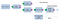Figure 1.17

The model’s loss function has three components, namely, confidence loss, coordinates loss, and classification loss. All components are considered to mean square error for calculating loss.

Then we backpropagate the error for weight adjustment.

Testing:

In the testing, we give the input image to the trained YOLO model, as shown in Figure 1.18. Then we get the output volume of dimension 19 X 19 X 5 X (5 + Number of classes), which contains 1805 bounding boxes because each anchor of each grid cell will predict the object with some probability. Hence, we have to eliminate the extra bounding boxes, which can be done through the non-max suppression algorithm. After removing unnecessary bounding box coordinates, we remain with necessary bounding box coordinates that have an object. We will draw the bounding box in the image for visual judgment.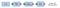Figure 1.18

YOLO Output:

Conclusion:

The YOLO method of object detection seems to be working best when considering the real-time object detection system. Comparing different object detection models was done mainly considering training and testing based on the COCO dataset. The real-time object detection can be merged with the other modules of, let say, an automated vehicle. In YOLO, we try to optimize the classification and localization error simultaneously. The fact behind the YOLO being fast is that the convolutional operation is performed only once on an image while the other methods perform several convolutional operations on a particular region. This, though, hampers the accuracy slightly but is a better tradeoff considering the time factor. YOLO works better when the objects in the image are not too small but occupies a considerable portion of the image. YOLO method works on the concept of anchor boxes, and thus determining the size and number of anchor boxes becomes crucial. We must determine the number of anchor boxes based on the variations of the object’s size and shape in the dataset. Thus, the anchor box guides YOLO methods, which help in detecting many objects in a particular grid cell. The overlapping objects can also be detected with the help of anchor boxes in YOLO. Many anchor boxes can be added to increase the accuracy of YOLO but can increase the inference time. YOLO also generalizes well to the new domain, making it ideal for the application that relies on fast and robust object detection.

References:

 Sermanet, Pierre & Eigen, David & Zhang, Xiang & Mathieu, Michael & Fergus, Rob & Lecun, Yann. (2013). OverFeat: Integrated Recognition, Localization and Detection using Convolutional Networks. International Conference on Learning Representations (ICLR)(Ban ).

 R. Girshick, J. Donahue, T. Darrell and J. Malik, “Rich Feature Hierarchies for Accurate Object Detection and Semantic Segmentation,” 2014 IEEE Conference on Computer Vision and Pattern Recognition, Columbus, OH, 2014, pp. 580587, doi: 10.1109/CVPR.2014.81.

 J. Uijlings, K. van de Sande, T. Gevers, and A. Smeulders. Selective search for object recognition. IJCV, 2013.

 R. Girshick, “Fast R-CNN,” 2015 IEEE International Conference on Computer Vision (ICCV), Santiago, 2015, pp. 1440–1448, do: 10.1109/ICCV.2015.169.

 S. Ren, K. He, R. Girshick and J. Sun, “Faster R-CNN: Towards Real-Time Object Detection with Region Proposal Networks,” in IEEE Transactions on Pattern Analysis and Machine Intelligence, vol. 39, no. 6, pp. 1137–1149, 1 June 2017, doi: 10.1109/TPAMI.2016.2577031.

 J. Redmon, S. Divvala, R. Girshick and A. Farhadi, “You Only Look Once: Unified, Real-Time Object Detection,” 2016 IEEE Conference on Computer Vision and Pattern Recognition (CVPR), Las Vegas, NV, 2016, pp. 779–788, do: 10.1109/CVPR.2016.91.

 J. Redmon and A. Farhadi, “YOLO9000: Better, Faster, Stronger,” 2017 IEEE Conference on Computer Vision and Pattern Recognition (CVPR), Honolulu, HI, 2017, pp. 6517–6525, doi: 10.1109/CVPR.2017.690.

 Redmon, Joseph & Farhadi, Ali. (2018). YOLOv3: An Incremental Improvement.

Special thanks to Rohit Agarwal and Shivani Saini for helping me in the Deep learning domain.

Indian Institute of Technology, Bhubaneswar| Passionate about Deep Learning| https://shobhiit.me/

## More from Shobhit

Indian Institute of Technology, Bhubaneswar| Passionate about Deep Learning| https://shobhiit.me/